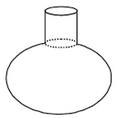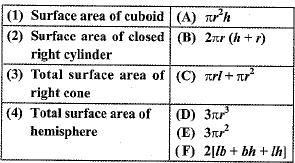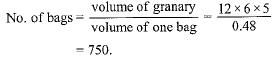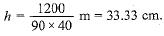Courses

# Important Questions: Surface Area And Volumes

## 10 Questions MCQ Test Mathematics (Maths) Class 10 | Important Questions: Surface Area And Volumes

Description
This mock test of Important Questions: Surface Area And Volumes for Class 10 helps you for every Class 10 entrance exam. This contains 10 Multiple Choice Questions for Class 10 Important Questions: Surface Area And Volumes (mcq) to study with solutions a complete question bank. The solved questions answers in this Important Questions: Surface Area And Volumes quiz give you a good mix of easy questions and tough questions. Class 10 students definitely take this Important Questions: Surface Area And Volumes exercise for a better result in the exam. You can find other Important Questions: Surface Area And Volumes extra questions, long questions & short questions for Class 10 on EduRev as well by searching above.
QUESTION: 1

Solution:
QUESTION: 2

### A surahi is the combination of

Solution:QUESTION: 3

### A cubical ice cream brick of edge 22 cm is to be distributed among some children by filling ice cream cones of radius 2 cm and height 7 cm upto its brim. How many children will get the ice cream cones?

Solution:
QUESTION: 4

Match the columnSolution:
QUESTION: 5

The volume of the largest right circular cone that can be cut out from a cube of edge 4.2 cm is

Solution:

The volume of the largest right circular cone that can be cut out from a cube of edge 4.2 cm is 19.404 cm3.

Edge of the cube = 4.2 cm

i.e 2r = 4.2

r = 4.2/2 = 2.1 cm

h = 4.2 cm

Volume of the cone = 1/3 * pi * r2 * h

=> 1/3 * 22/7 * 2.1 * 2.1 * 4.2

=> 19.404 cm3

Hence, the volume of the largest right circular cone that can be cut out from a cube of edge 4.2 cm is 19.404 cm3.

QUESTION: 6

The radius (in cm) of the largest right circular cone that can be cut out from a cube of edge 4.2 cm is

Solution:

Edge of the cube = 4.2 cm
Diameter of base of largest possible cone = 4.2 cm
∴ Radius = 4.2/2 = 2.1 cm

QUESTION: 7

A shuttle cock used for playing badminton has the shape of the combination of

Solution:
QUESTION: 8

How many bags of grain can be stored in a cuboid granary 12 m x 6 m x 5 m. If each bag occupies a space of 0.48 m3 ?

Solution:QUESTION: 9

In a swimming pool measuring 90 m x 40 m, 150 men take a dip. If the average displacement of water by a man is 8 m3, then rise in water level is

Solution:

Volume of water displaced = 150 x 8 = 1200 m3
⇒ 90 x 40 x h = 1200
⇒QUESTION: 10

A solid piece of iron in the form of a cuboid of dimensions 49 cm x 33 cm x 24 cm, is moulded to form a solid sphere. The radius of the sphere is

Solution: